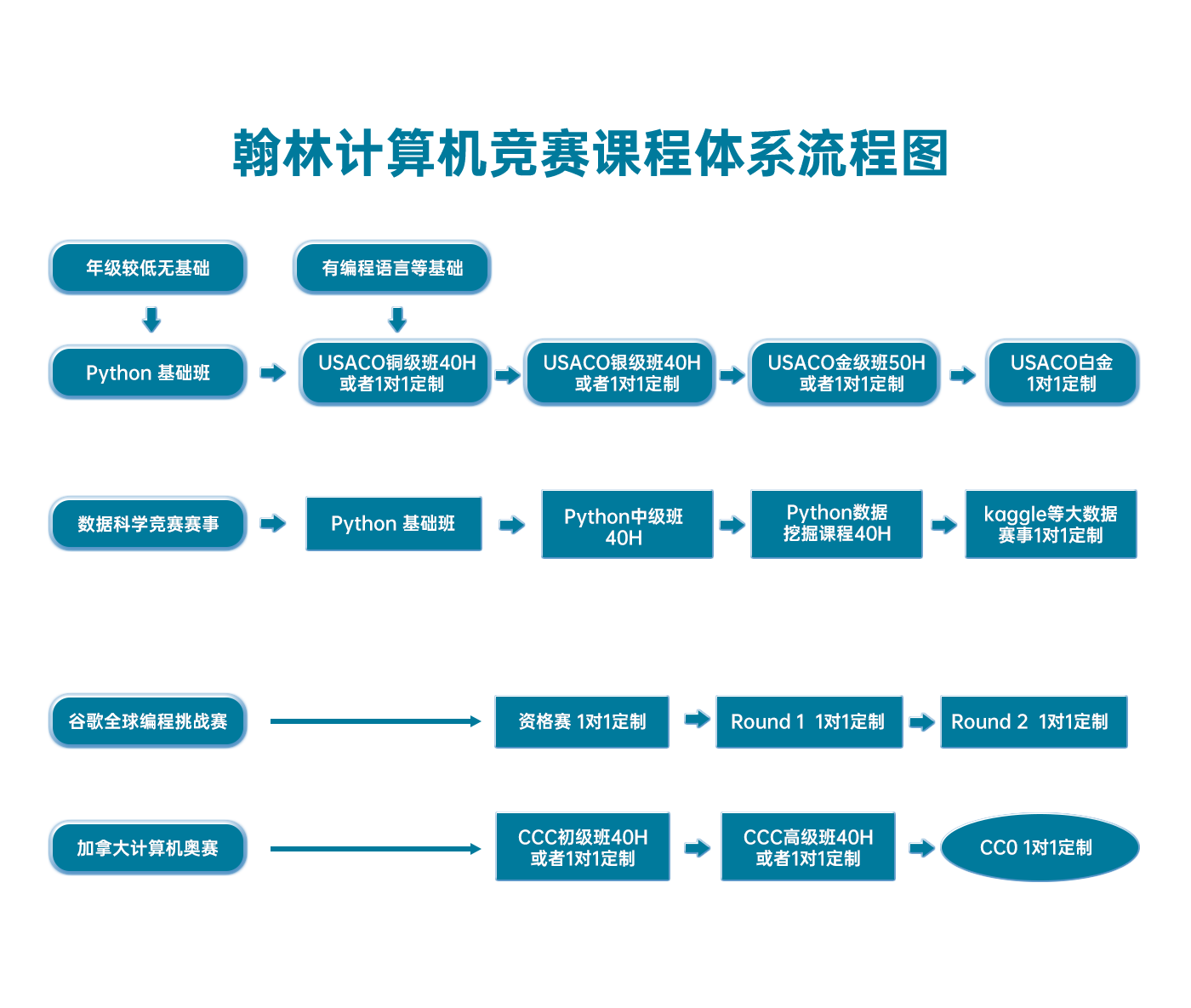# USACO 2015 US Open, Gold Problem 1. Googol

USACO2015OPEN-G1

(Analysis by Steven Hao)

We first present a naive algorithm that recursively iterates through all nodes.

Below is Python code implementing this naive algorithm, which we will soon optimize.

import sys

print cur
sys.stdout.flush()
return [int(_) for _ in raw_input().split()]

def count(cur): # count size of subtree at cur
if cur == 0:
return 0
rgt = count(b)
lft = count(a)
return 1 + lft + rgt

ans = count(1)


Of course, with N10^100, this code is too slow!

We will make an optimization that makes use of the fact lftrgt{0,1}, where lft and rgt are the size of the left and right subtree of cur (as used in the above code).

We do not need to compute lft from scratch; we merely need to compute it given that it is either rgt or rgt+1.

In other words, we evaluate lft=countGiven(a,rgt).

When computing countGiven(cur,x), we use the same approach as before: compute the size of the left subtree of cur and the right subtree of cur (which we shall again name lft and rgt), then evaluate 1+lft+rgt to find the size of the subtree at cur.

In general, if lft+rgt=z, then lft=z/2and rgt=z/2. This is very useful, as we know z+1 is either x or x+1.

If xx is even, then lft{⌈(x1)/2,x/2}lft=x/2.

If xx is odd, then rgt{⌊(x1)/2,x/2}rgt=(x1)/2.

Knowing lft lets us recursively find rgt=countGiven(b,lft1), and vice versa -- lft=countGiven(a,rgt). Overall, this means we only need to recurse once to count the size of a tree given that its size is one of two options.

Below is code implementing this optimization.

def countGiven(cur, x): # given ret = x or ret = x + 1, find ret
if cur == 0:
return 0

# lft + rgt = x - 1 or lft + rgt = x
if x % 2 == 1:
rgt = (x - 1) / 2
lft = countGiven(a, rgt)
return 1 + lft + rgt
else:
lft = x / 2
rgt = countGiven(b, lft - 1)
return 1 + lft + rgt

def count(cur): # count size of subtree at cur
if cur == 0:
return 0
rgt = count(b)
lft = countGiven(a, rgt)
return 1 + lft + rgt


We can analyze the number of queries this code makes -- we want it to be less than the allowed 70,000.

In the maximum case, N=10^100. Then the height H≈Lg2N=100Lg210<10010/3333

The procedure countcount is called once for each level. At a height hhcountcount spawns a countGivencountGiven which makes hh queries, so overall, there are 1+2++33310^6/18<70000 queries.

[Note from problem author: Trees of this sort are sometimes called "Braun trees" in the computing literature; they have a number of nice uses particularly in "functional" programming languages, since they are easy to manipulate in a recursive context. For example, to insert a new element in O(logn) time while maintaining the balancing condition, we can just insert it recursively into our left subtree and then swap the left and right children of the root.]

### 翰林USACO课程体系流程图